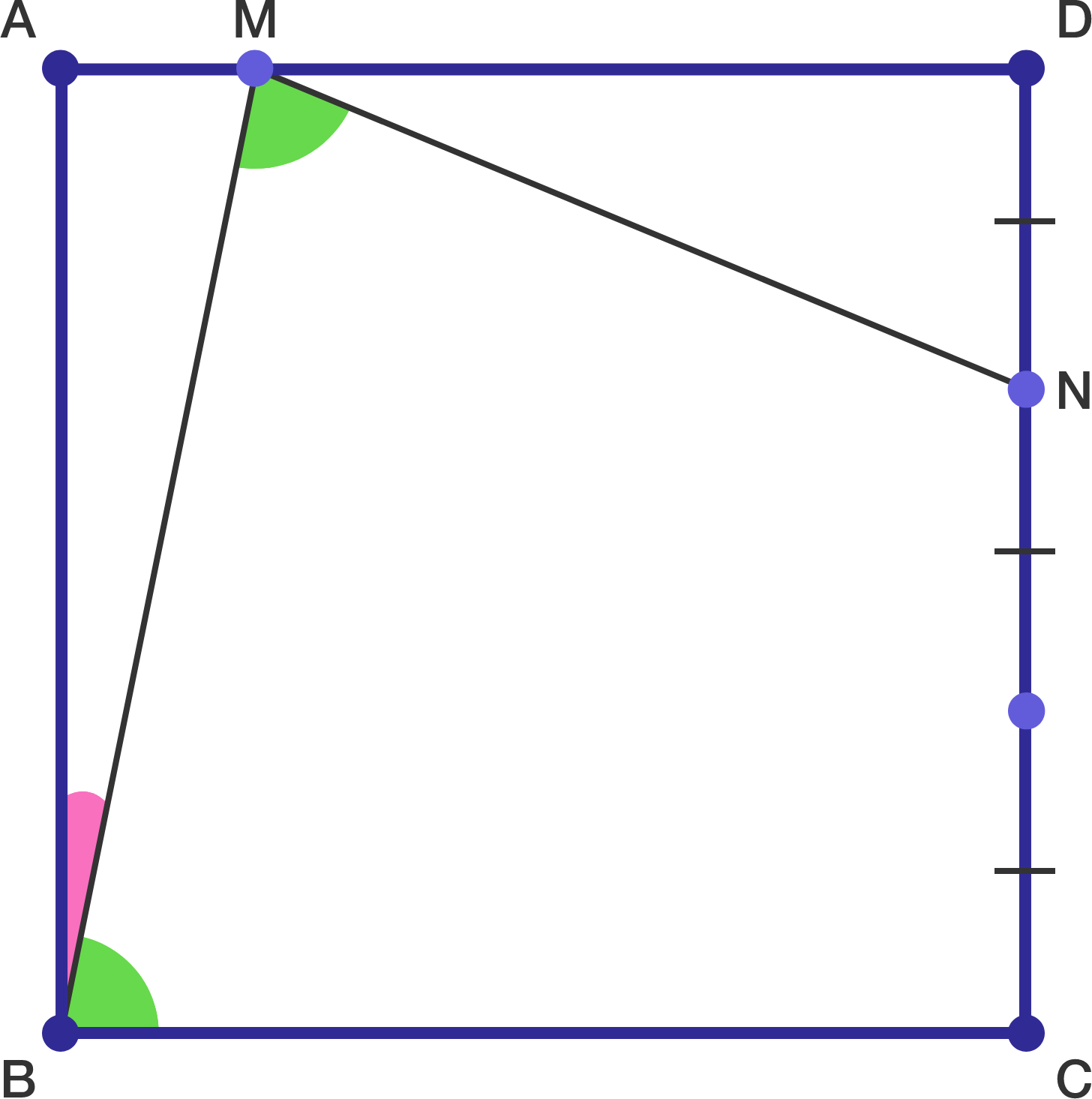# Squares are <3

Geometry Level 3In the diagram above, $ABCD$ is a square, $NC = 2DN,$ and $\angle NMB = \angle MBC.$

If $MDN$ is not a degenerate triangle (that is, its area is non-zero), what is $\tan \angle ABM?$

×

Problem Loading...

Note Loading...

Set Loading...